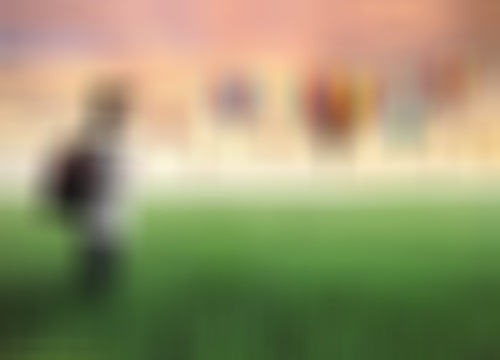# OpenCV - Blur (Averaging)

Blurring (smoothing) is the commonly used image processing operation for reducing the image noise. The process removes high-frequency content, like edges, from the image and makes it smooth.

In general blurring is achieved by convolving (each element of the image is added to its local neighbors, weighted by the kernel) the image through a low pass filter kernel.

## Blur (Averaging)

During this operation, the image is convolved with a box filter (normalized). In this process, the central element of the image is replaced by the average of all the pixels in the kernel area.

You can perform this operation on an image using the method blur() of the imgproc class. Following is the syntax of this method −

```blur(src, dst, ksize, anchor, borderType)
```

This method accepts the following parameters −

• src − A Mat object representing the source (input image) for this operation.

• dst − A Mat object representing the destination (output image) for this operation.

• ksize − A Size object representing the size of the kernel.

• anchor − A variable of the type integer representing the anchor point.

• borderType − A variable of the type integer representing the type of the border to be used to the output.

## Example

The following program demonstrates how to perform the averaging (blur) operation on an image.

```import org.opencv.core.Core;
import org.opencv.core.Mat;
import org.opencv.core.Point;
import org.opencv.core.Size;
import org.opencv.imgcodecs.Imgcodecs;
import org.opencv.imgproc.Imgproc;

public class BlurTest {
public static void main(String args[]) {

// Reading the Image from the file and storing it in to a Matrix object
String file ="C:/EXAMPLES/OpenCV/sample.jpg";

// Creating an empty matrix to store the result
Mat dst = new Mat();

// Creating the Size and Point objects
Size size = new Size(45, 45);
Point point = new Point(20, 30);

// Applying Blur effect on the Image
Imgproc.blur(src, dst, size, point, Core.BORDER_DEFAULT);

// blur(Mat src, Mat dst, Size ksize, Point anchor, int borderType)
// Writing the image
Imgcodecs.imwrite("E:/OpenCV/chap9/blur.jpg", dst);
System.out.println("Image processed");
}
}
```

Assume that following is the input image sample.jpg specified in the above program.## Output

On executing the program, you will get the following output −

```Image Processed
```

If you open the specified path, you can observe the output image as follows −